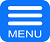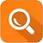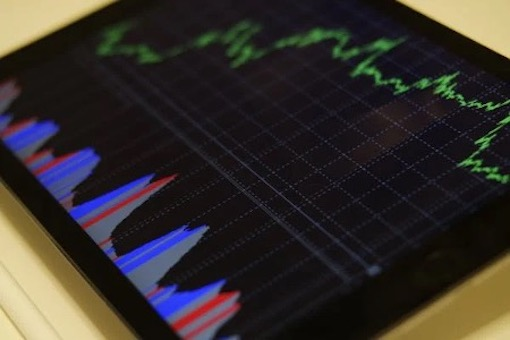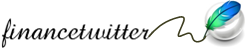### 8 Trading Tips You Will Want To HearAugust 19th, 2022 by financetwitter

1. Triple bottom pattern

The triple bottom pattern is a bullish reversal pattern that is created when an asset price hits a support level three times and then starts to rebound higher. This pattern can be found in any time frame but is most commonly seen on longer-term charts. Also, to identify the triple bottom pattern, we need to make sure that there is a significant distance between the three lows. It’s important to note that the triple bottom pattern is not complete until the asset price breaks above the resistance level created by the three lows.

2. MACD

The MACD (Moving Average Convergence Divergence) is a technical indicator that is used to measure the momentum of an asset price. The MACD is calculated by subtracting the 26-period Exponential Moving Average (EMA) from the 12-period EMA. A buy signal is generated when the MACD line crosses above the signal line, while a sell signal is generated when the MACD line crosses below the signal line. Additionally, the MACD histogram can be used to generate buy and sell signals. A buy signal is generated when the MACD histogram turns positive, while a sell signal is generated when the MACD histogram turns negative.3. Relative Strength Index (RSI)

The RSI (Relative Strength Index) is a technical indicator that measures the speed and change of an asset price. The RSI is calculated by dividing the average gain of the asset price over a certain period by the average loss over that same period. The RSI is considered to be overbought when it is above 70 and oversold when it is below 30. A buy signal is generated when the RSI crosses above the oversold level, while a sell signal is generated when the RSI crosses below the overbought level.

4. Fibonacci Retracement Levels

Fibonacci retracement levels are horizontal lines that are used to identify possible support and resistance levels for an asset price. These levels are calculated by taking the highest high and lowest low of an asset price and then dividing that range by the Fibonacci ratios of 23.6%, 38.2%, 50%, 61.8%, and 100%. The 23.6% and 38.2% levels are considered to be the most important support and resistance levels, while the 61.8% level is considered to be the most important Fibonacci retracement level.

5. Pivot Points

Pivot points are technical indicators that are used to identify possible support and resistance levels for an asset price. Pivot points are calculated by taking the average of the high, low, and close of an asset price over a certain period of time. The most common time frames used to calculate pivot points are daily, weekly, and monthly. Additionally, there are three types of pivot points: standard, Fibonacci, and Camarilla. Standard pivot points use a simple average of the high, low, and close, while Fibonacci pivot points use the Fibonacci ratios of 23.6%, 38.2%, and 61.8% to calculate the support and resistance levels. Camarilla pivot points use a different set of Fibonacci ratios, which are listed below.6. Bollinger Bands

Bollinger bands are technical indicators that are used to measure the volatility of an asset price. Bollinger bands are calculated by taking the standard deviation of an asset price over a certain period of time and then adding and subtracting that value from the asset’s moving average. The upper Bollinger band is considered to be a resistance level, while the lower Bollinger band is considered to be a support level. Additionally, Bollinger bands can be used to generate buy and sell signals. A buy signal is generated when the asset price crosses above the upper Bollinger band, while a sell signal is generated when the asset price crosses below the lower Bollinger band.

7. Candlestick Patterns

Candlestick patterns are technical indicators that are used to predict the future direction of an asset price. There are many different candlestick patterns, but some of the most common ones are: The hammer candlestick pattern is a bullish reversal pattern that is formed when the asset price falls and then rallies to close near its opening price. The long lower shadow indicates that there was strong selling pressure during the period, while the short upper shadow indicates that there was strong buying pressure near the end of the period. The engulfing candlestick pattern is a bullish reversal pattern that is formed when the asset price falls and then rallies to close above the opening price of the previous period.

8. Moving Averages

Moving averages are technical indicators that are used to smooth out the price action of an asset and to identify the trend. Moving averages are calculated by taking the average of an asset’s price over a certain period of time. The most common time frames used to calculate moving averages are 20, 50, 100, and 200 periods. Additionally, there are two types of moving averages: simple moving averages (SMAs) and exponential moving averages (EMAs). SMAs are calculated by simply taking the average of an asset’s price over a certain period of time, while EMAs are calculated by giving more weight to the recent data points.

There you have it! These are eight important trading tips that you will want to hear. Remember, it is important to do your own research and to test out different technical indicators before making any real-world trades. It’s also important to have a solid risk management strategy in place before you begin trading. But if you follow these tips, you’ll be well on your way to becoming a successful trader.If you enjoyed this post, what shall you do next? Consider: